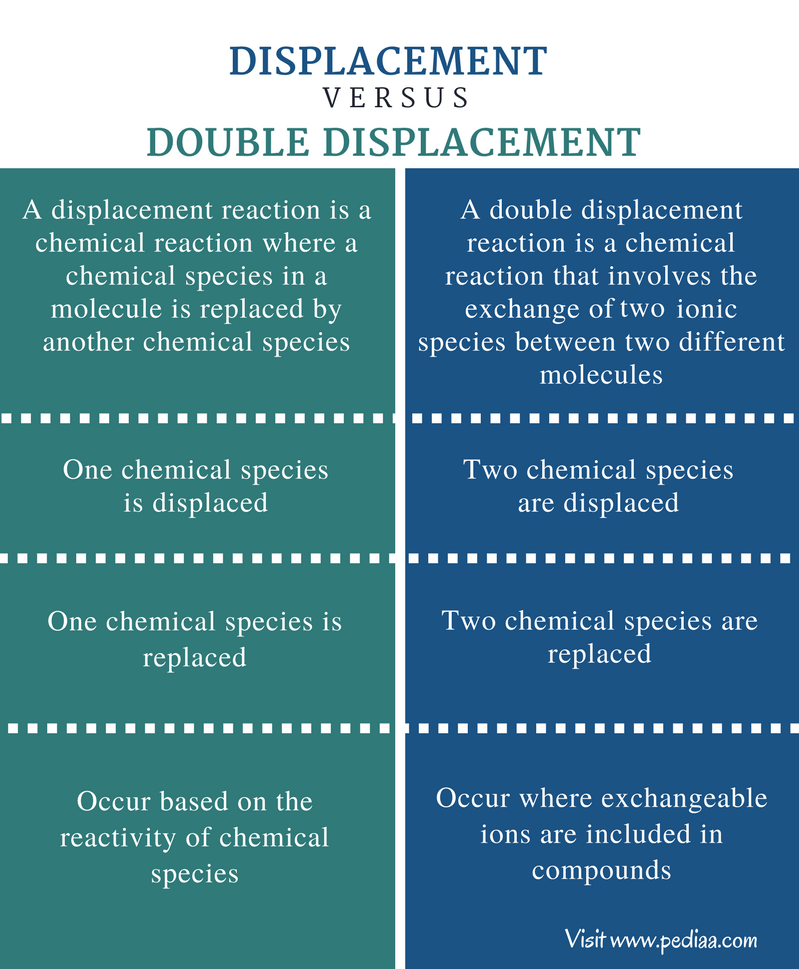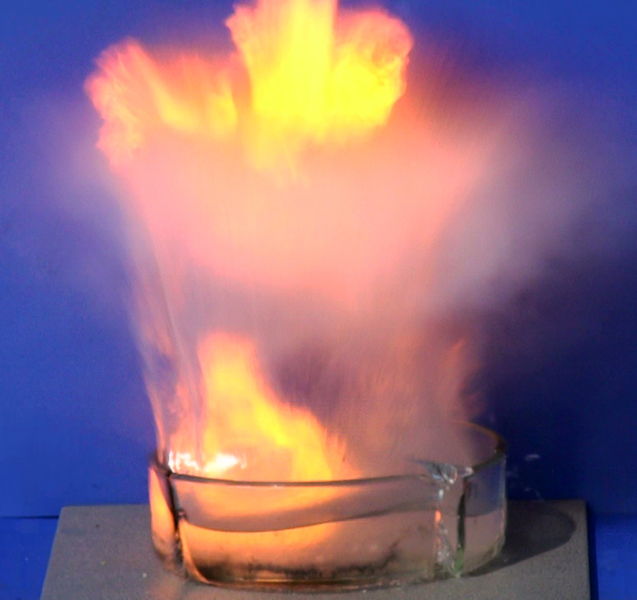# Difference Between Displacement and Double Displacement Reaction

## Main Difference – Displacement vs Double Displacement Reaction

Displacement reactions occur in the presence of a reactive chemical species. A very reactive chemical species can replace an anion or a cation of a compound. This displacement occurs according to the reactivity series. The reactivity series is a list of elements that shows the order of the reactivity of those elements from most reactive elements to the least reactive elements. Therefore, it gives an idea about the capability of an element to displace another element. This displacement can occur as a single displacement or a double displacement. The main difference between displacement and double displacement reaction is that in displacement reaction, a single chemical species is replaced whereas, in double displacement reactions, two chemical species are replaced.

### Key Areas Covered

1. What is Displacement Reaction
– Definition, Properties, Examples
2. What is Double Displacement Reaction
– Definition, Properties, Examples
3. What is the Difference Between Displacement and Double Displacement Reaction
– Comparison of Key Differences

Key Terms: Acid-Base Reactions, Alkylation, Displacement Reaction, Double Displacement Reaction, Neutralization, Precipitation, Reactivity Series## What is a Displacement Reaction

A displacement reaction is a chemical reaction that occurs by replacing a chemical species in a molecule by another chemical species. Displacement reactions take place in the presence of a more reactive species which has a high binding affinity for a cation or an anion present in a compound. The reactive species can be a metal element, anion or a cation. The general form of a displacement reaction can be given as below.

A-B    +    C      →       A-C      +    B

In the above reaction, B in the A-B molecule has been replaced by C. Therefore, C is a highly reactive chemical species than B. In order to complete the displacement reaction, A should have binding affinity for both B and C. B and C can be a metal element, anion or a cation.

The elements that are displaced can be predicted by looking at the reactivity series. The reactivity series is a list of elements that is categorized in the order of their reactivity from the most reactive element to the least reactive element. Therefore, elements in the upper part of that list are highly reactive than the elements in the lower part of the list. Then the elements that are in the lower part can be replaced by the elements in the upper part easily.

Zn(s)    +     2HCl(aq)       →         ZnCl2(aq)     +       H2(g)

The above reaction shows the displacement of H+ cation by Zn metal. Here the Zn is bonded to Cl anion.

The displacement reactions are used commonly in displacing halogens from compounds. The order of halogens in the reactivity series is F>Cl>Br>I. This means the highest reactivity is shown by Fluorine whereas the lowest reactivity is shown by iodine. Therefore, if we need to obtain Br2 liquid out of NaBr solution, we can bubble Cl2(g) and use a separation method to separate bromine liquid. The reaction is given below.

NaBr(aq)      +        Cl2(g)           →              NaCl(aq)         +          Br2(aq)

If least reactive elements are added to a compound, no displacement will occur. For example, gold (Au) is not a very reactive metal, so it does not react with even some acids like HCl.Figure 1: Reaction between sodium (Na) and water

When sodium is added to water, it shows an exploding reaction. That is due to the high reactivity of sodium. Sodium reacts with water and form Sodium hydroxide (NaOH) and hydrogen gas (H2). There, Sodium replaces the H+ from H-OH (water molecule).

## What is a Double Displacement Reaction

A double displacement reaction is a chemical reaction that involves the exchange of two ionic species between two different molecules. The formed product is a new compound which can be either a liquid or a solid. The general form of this kind of reactions is given below.

P-Q     +     R-S            →            P-S          +           R-Q

The bonds between ions of reactants are broken and new bonds are formed. These bonds can be either ionic bonds or covalent bonds. Some precipitation reactions, acid-base reactions, alkylation reactions are good examples for double displacement reactions.

Li2SO4(aq)   +    BaCl2(aq)       →        2LiCl(aq)      +       BaSO4(s)

If we want to take Barium out of a solution, we can just add Lithium Sulfate. Then Barium Sulfate (BaSO4), which is a white precipitate, will be formed. Here, the SO42- ion has been exchanged with Cl ions of Barium Chloride (BaCl2). Most double displacement reactions form precipitates.Figure 2: Neutralization reaction between sodium hydroxide and hydrochloric acid

These double displacement reactions are also common in acid-base reactions. They are called neutralization reactions. For example, the reaction between NaOH (sodium hydroxide) and HCl (hydrochloric acid) is a neutralization reaction where a double displacement occurs.

NaOH(aq)    +      HCl(aq)          →          NaCl(aq)      +        H2O(l)

In the above reaction, Cl- ion is exchanged with OH- ion. The new products are sodium chloride (NaCl) and a water molecule (H2O).

## Difference Between Displacement and Double Displacement Reaction

### Definition

Displacement Reaction: A displacement reaction is a chemical reaction where a chemical species in a molecule is replaced by another chemical species.

Double Displacement Reaction: A double displacement reaction is a chemical reaction that involves the exchange of two ionic species between two different molecules.

### Displacement

Displacement Reaction: In displacement reactions, one chemical species is displaced.

Double Displacement Reaction: In double displacement reactions, two chemical species are displaced.

### Replacement

Displacement Reaction: In displacement reactions, one chemical species is replaced.

Double Displacement Reaction: In double displacement reactions, two chemical species are replaced.

### Reactivity

Displacement Reaction: Displacement reactions occur based on the reactivity of chemical species.

Double Displacement Reaction: Double displacement reactions occur where exchangeable ions are included in compounds.

### Conclusion

Double displacement reactions are a type of displacement reactions. In both reactions, a displacement and a replacement occur at the same time. The main difference between displacement and double displacement reaction is that in displacement reaction, a single chemical species is replaced whereas in double displacement reactions, two chemical species are replaced.

##### References:

1. Helmenstine, Anne Marie. “What Is a Double Displacement Reaction in Chemistry?” ThoughtCo. N.p., n.d. Web. Available here. 24 July 2017.
2.”Double Displacement reaction .” Study.com. Study.com, n.d. Web. Available here. 24 July 2017.

##### Image Courtesy:

1. “Neutralization reaction between sodium hydroxide and hydrochloric acid” By Bluescan sv.wiki – Own work (CC BY-SA 3.0) via Commons Wikimedia
2. “Sodium and Water”By Naatriumi_reaktsioon_veega_purustab_klaasist_anuma.jpg: Tavoromannderivative work: Tony Mach – This file was derived fromNaatriumi reaktsioon veega purustab klaasist anuma.jpg: (CC BY-SA 3.0) via Commons Wikimedia